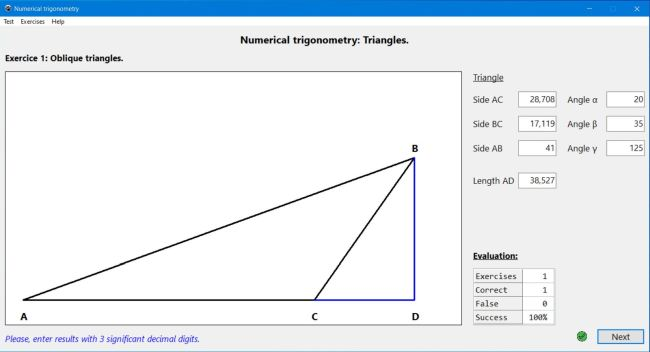# Computing: Free Pascal Programming

 Programming Home Home Contact

## Numerical trigonometry: Triangles (Trigonometry1).

Description: Mathematics trainer (exercise generator), dealing with numerical trigonometry, or more precisely with triangles. 5 types of exercises are considered:

• The Pythagorean theorem
• Similar right triangles
• Trigonometric ratios
• Oblique triangles
• The law of sines
The application draws the triangle(s) and gives some of the values (e.g. the lengths of 2 sides, with the Pythagorean theorem) and the user has to calculate the other one(s), the different exercise types being individually selectable, so the student can test her knowledge for specific topics.

Possible extensions: Exercises, mixing the different types of calculatons.

Free Pascal features: Showing/hiding/modifying controls, depending on actual situation. Drawing geometrical shapes, using canvas.

Screenshots: